#Choosing the Right Metric for Evaluating Machine Learning Models  –  Part 1

Each machine learning model is trying to solve a problem with a different objective using a different dataset and hence, it is important to understand the context before choosing a metric.comments

By Alvira Swalin, University of San Francisco

In the world of postmodernism, Relativism has been, in its various guises, both one of the most popular and most reviled philosophical doctrines. According to Relativismthere is no universal and objective truth; rather each point of view has its own truth. You must be wondering why I am discussing it and how it is even related to Data Science.

Well, in this post, I will be discussing the usefulness of each error metric depending on the objective and the problem we are trying to solve. When someone tells you that “USA is the best country”, the first question that you should ask is on what basis is this statement being made. Are we judging each country on the basis of their economic status, or their health facilities etc.? Similarly each machine learning model is trying to solve a problem with a different objective using a different dataset and hence, it is important to understand the context before choosing a metric.

### Most Useful Metrics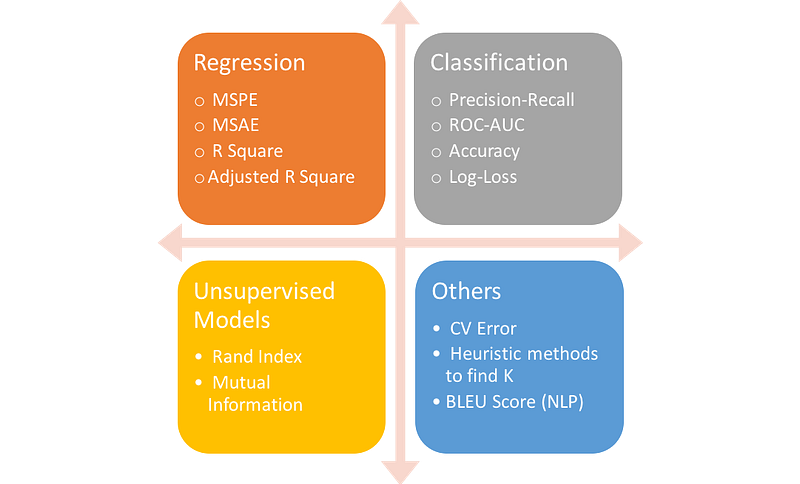In the first blog, we will cover metrics in regression only.

### Regression Metrics

Most of the blogs have focussed on classification metrics like precision, recall, AUC etc. For a change, I wanted to explore all kinds of metrics including those used in regression as well. MAE and RMSE are the two most popular metrics for continuous variables. Let’s start with the more popular one.

RMSE (Root Mean Square Error)

It represents the sample standard deviation of the differences between predicted values and observed values (called residuals). Mathematically, it is calculated using this formula: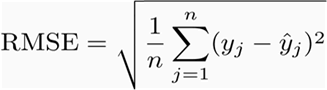MAE

MAE is the average of the absolute difference between the predicted values and observed value. The MAE is a linear score which means that all the individual differences are weighted equally in the average. For example, the difference between 10 and 0 will be twice the difference between 5 and 0. However, same is not true for RMSE which we will discuss more in details further. Mathematically, it is calculated using this formula: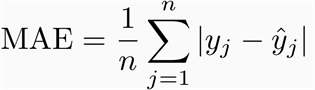So which one should you choose and why?

Well, it is easy to understand and interpret MAE because it directly takes the average of offsets whereas RMSE penalizes the higher difference more than MAE.

Let’s understand the above statement with the two examples:

Case 1: Actual Values = [2,4,6,8] , Predicted Values = [4,6,8,10]
Case 2: Actual Values = [2,4,6,8] , Predicted Values = [4,6,8,12]

MAE for case 1 = 2.0, RMSE for case 1 = 2.0
MAE for case 2 = 2.5, RMSE for case 2 = 2.65

From the above example, we can see that RMSE penalizes the last value prediction more heavily than MAE. Generally, RMSE will be higher than or equal to MAE. The only case where it equals MAE is when all the differences are equal or zero (true for case 1 where the difference between actual and predicted is 2 for all observations).

However, even after being more complex and biased towards higher deviation, RMSE is still the default metric of many models because loss function defined in terms of RMSE is smoothly differentiable and makes it easier to perform mathematical operations.

Though this may not sound very pleasing, it is a very important reason and makes it very popular. I will try to explain the above logic mathematically.

Let’s take a simple linear model in one variable: y = mx+b

Here, we are trying to find “m” and “b” and we are provided with data (x,y).

If we define loss function (J) in terms of RMSE: then we can easily differentiate J wrt. to m and b and get the updated m and b (this is how gradient descent works, I won’t be explaining it here)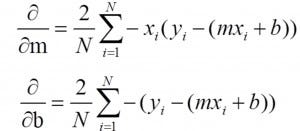The above equations are simpler to solve and the same won’t apply for MAE.

However if you want a metric just to compare between two models from interpretation point of view, then I think MAE is a better choice. It is important to note that the units of both RMSE & MAE are same as y values which is not true for R Square. The range of RMSE & MAE is from 0 to infinity.

Edit: One important distinction between MAE & RMSE that I forgot to mention earlier is that minimizing the squared error over a set of numbers results in finding its mean, and minimizing the absolute error results in finding its median. This is the reason why MAE is robust to outliers whereas RMSE is not. This answer explains this concept in detail.

R Squared (R²) and Adjusted R Squared

R Squared & Adjusted R Squared are often used for explanatory purposes and explains how well your selected independent variable(s) explain the variability in your dependent variable(s). Both these metrics are quite misunderstood and therefore I would like to clarify them first before going through their pros and cons.

Mathematically, R_Squared is given by: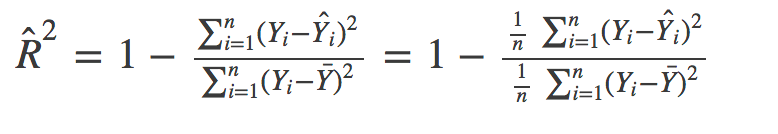The numerator is MSE ( average of the squares of the residuals) and the denominator is the variance in Y values. Higher the MSE, smaller the R_squared and poorer is the model.

Just like R², adjusted R² also shows how well terms fit a curve or line but adjusts for the number of terms in a model. It is given by below formula: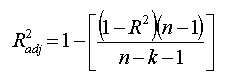where n is the total number of observations and k is the number of predictors. Adjusted R² will always be less than or equal to R²

Why should you choose Adjusted R² over R²?

There are some problems with normal R² which are solved by Adjusted R². An adjusted R² will consider the marginal improvement added by an additional term in your model. So it will increase if you add the useful terms and it will decrease if you add less useful predictors. However, R² increases with increasing terms even though the model is not actually improving. It will be easier to understand this with an example.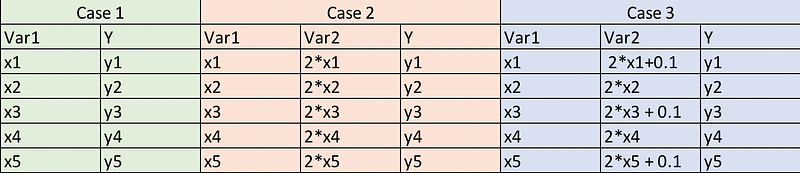Here, Case 1 is the simple case where we have 5 observations of (x,y). In case 2, we have one more variable which is twice of variable 1 (perfectly correlated with var 1). In Case 3, we have produced a slight disturbance in var2 such that it is no longer perfectly correlated with var1.

So if we fit simple ordinary least squares (OLS) model for each case, logically we are not providing any extra or useful information to case 2 and case 3 with respect to case 1. So our metric value should not improve for these models. However, it is actually not true for R² which gives a higher value for model 2 and 3. But your adjusted R² takes care of this problem and it is actually decreasing for both cases 2 & 3. Let’s give some numbers to these variables (x,y) and look at the results obtained in Python.

Note: Predicted values will be same for both model 1 and model 2 and therefore, r_squared will also be same because it depends only on predicted and actual values.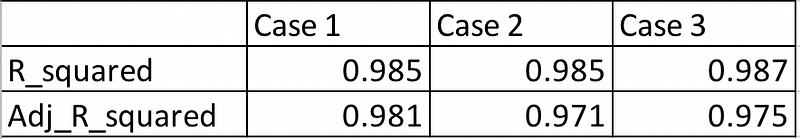From the above table, we can see that even though we are not adding any additional information from case 1 to case 2, still R² is increasing whereas adjusted R² is showing the correct trend (penalizing model 2 for more number of variables)

Comparison of Adjusted R² over RMSE

For the previous example, we will see that RMSE is same for case 1 and case 2 similar to R². This is the case where Adjusted R² does a better job than RMSE whose scope is limited to comparing predicted values with actual values. Also, the absolute value of RMSE does not actually tell how bad a model is. It can only be used to compare across two models whereas Adjusted R² easily does that. For example, if a model has adjusted R² equal to 0.05 then it is definitely poor.

However, if you care only about prediction accuracy then RMSE is best. It is computationally simple, easily differentiable and present as default metric for most of the models.

Common Misconception: I have often seen on the web that the range of R² lies between 0 and 1 which is not actually true. The maximum value of R² is 1 but minimum can be negative infinity. Consider the case where model is predicting highly negative value for all the observations even though y_actual is positive. In this case, R² will be less than 0. This will be a highly unlikely scenario but the possibility still exists.

### Bonus!

Here is an interesting metric to know about if you are interested in NLP and you deserve it for reaching the end. I recently got to know about it in Andrew Ng Deep Learning course and found it worth sharing.

BLEU (Bilingual Evaluation Understudy)

It is mostly used to measure the quality of machine translation with respect to the human translation. It uses a modified form of precision metric.

Steps to compute BLEU score:
1. Convert the sentence into unigrams, bigrams, trigrams, and 4-grams
2. Compute precision for n-grams of size 1 to 4
3. Take the exponential of the weighted average of all those precision values
4. Multiply it with brevity penalty (will explain later)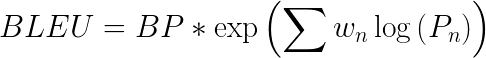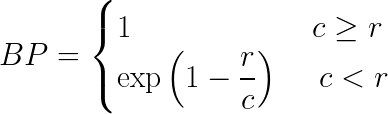Here BP is the brevity penalty, r & c is the number of words in reference & candidate respectively, w — weights, P — Precision values

Example:

Reference: The cat is sitting on the mat
Machine Translation 1: On the mat is a cat
Machine Translation 2: There is cat sitting cat

Let’s compare the above two translations with BLEU score.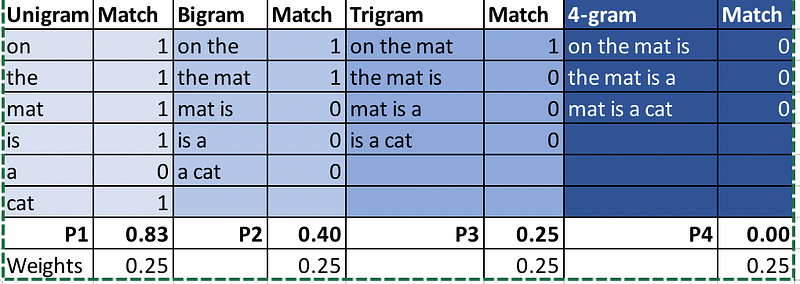Here I am using nltk.translate.bleu_score package -

Final Results: BLEU (MT1) = 0.454, BLEU (MT2) = 0.59

Why do we add brevity penalty?

Brevity Penalty penalizes candidates shorter than their reference translations. For example, if the candidate for the above mentioned reference is “ The cat”, then it will have a high precision for unigram and bigram because, both the words are present in the reference in the same order. However, the length is too short and does not actually reflect the meaning of reference.

With this brevity penalty in place, a high-scoring candidate translation must now match the reference in terms of length, same words and order of words.

Hopefully, most of the useful measures in regression are covered in this blog. Please comment if anything is missed, will really appreciate ideas for the next blog. I will discuss the important metrics of classification and unsupervised learning in the next blog. Stay tuned! In the meantime, check out my other blogs here!

References

Bio: Alvira Swalin (Medium) is currently pursuing Master's in Data Science at USF, I am particularly interested in Machine Learning & Predictive Modeling. She is a Data Science Intern at Price (Fx).

Original. Reposted with permission.

Related: